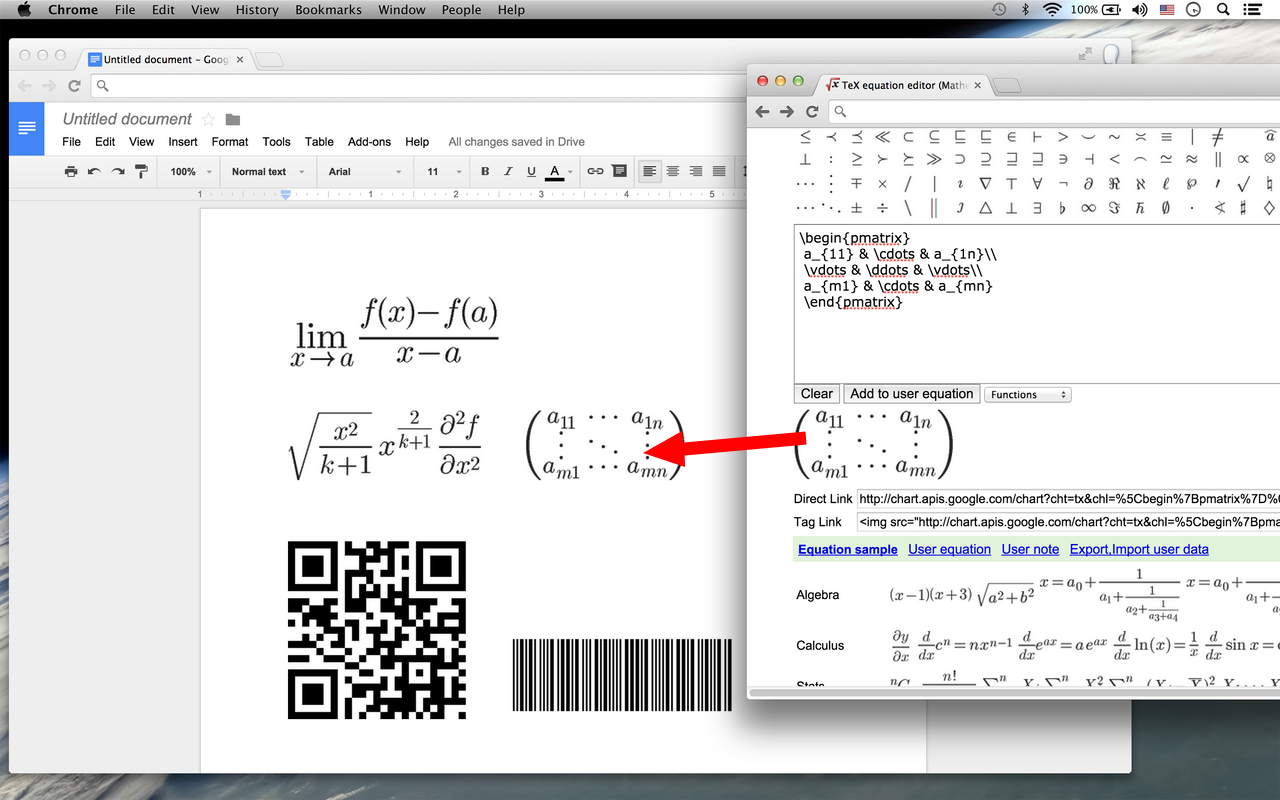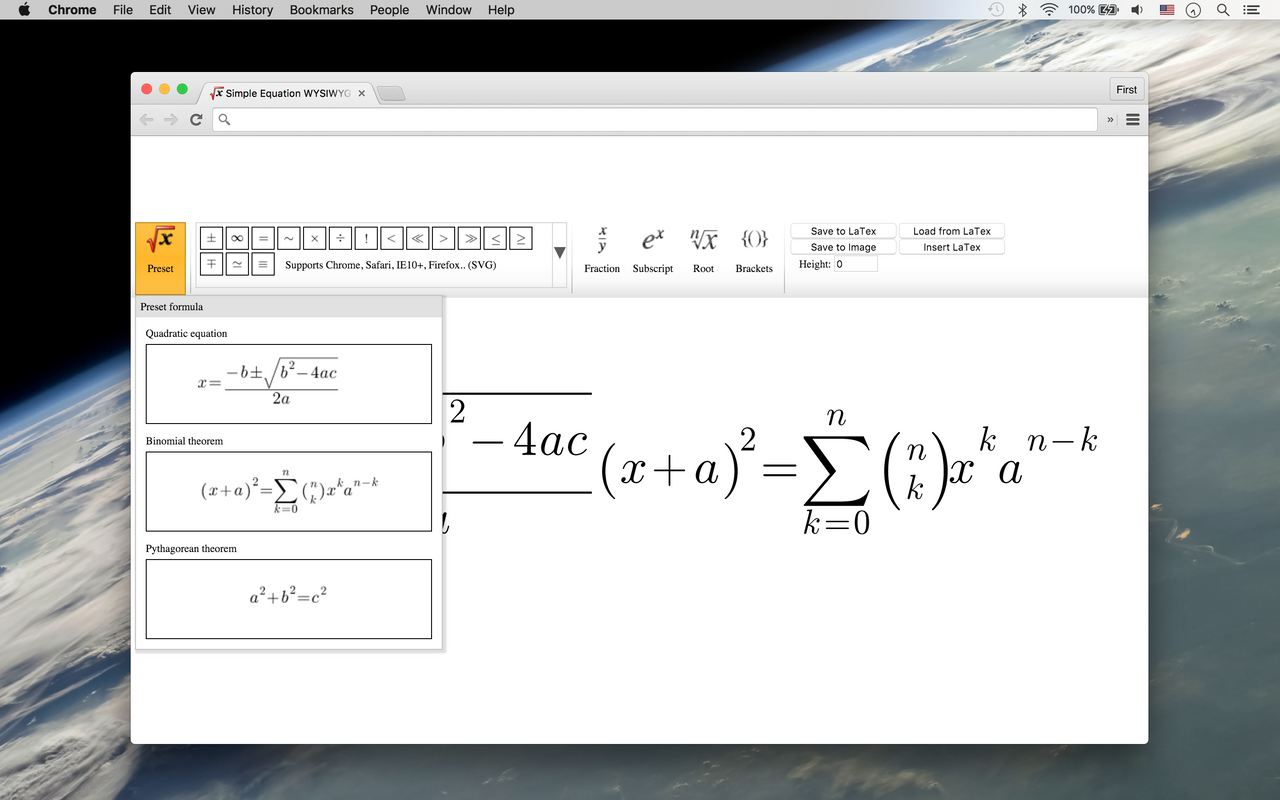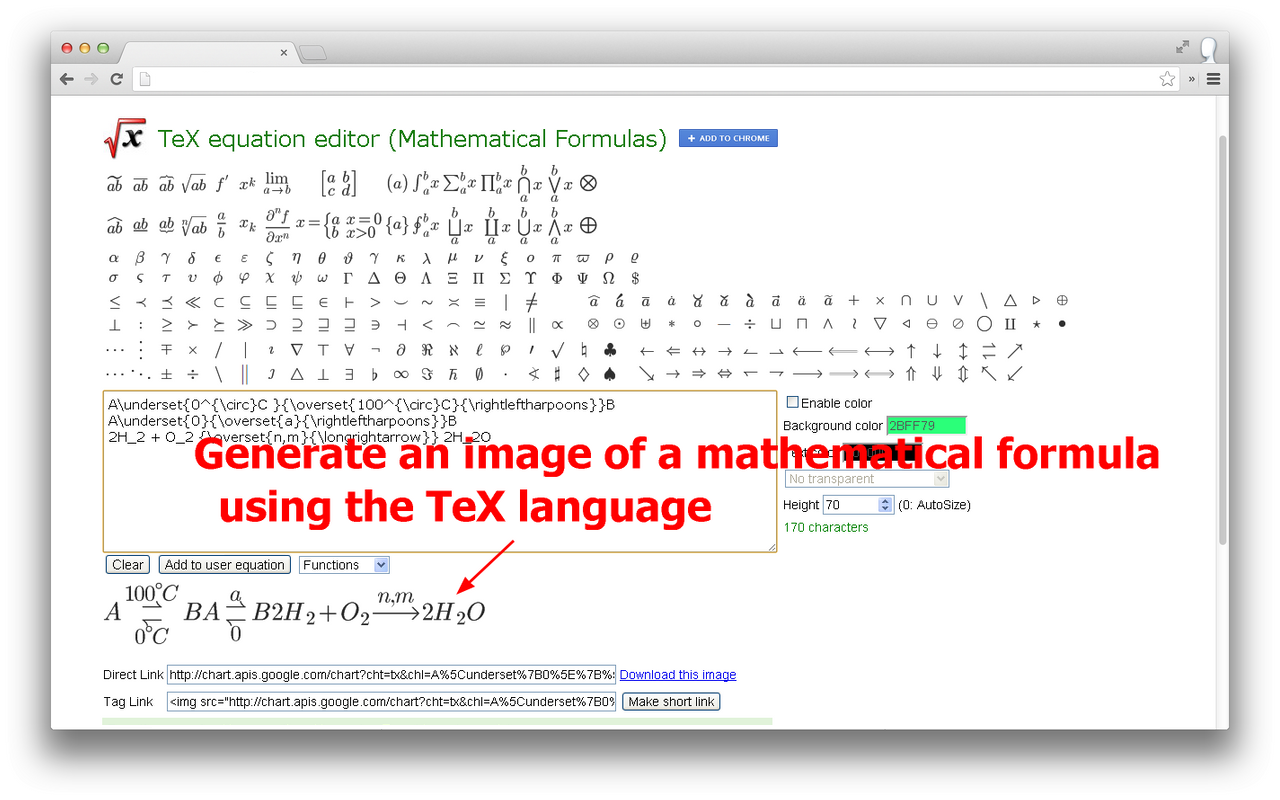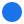You can generate an image of a mathematical formula using the TeX. Paste the TeX equation, QR Code, Barcode to Google documents.
Listing updated:March 15, 2022
Works with:
33M+Overview
```This app is an equation editor for writing math equations, expressions, mathematical characters, and operations. Supports realtime TeX code rendering.
You can also generate an image of a mathematical formula using the TeX(or LaTeX) language.
This is useful for displaying complex formulas on your web page.
Provides connect with Google Drive. Paste the TeX equation, QR Code, Barcode to Google documents.

TeX equation editor that creates graphical equations. Produces code for directly embedding equations into HTML websites, forums or blogs. Images may also be dragged into other applications like Word.
You can change the equation background color, text color, transparency, size.```
PricingFree of charge
DeveloperSee your primary Google Account email addressSee your personal info, including any personal info you've made publicly available